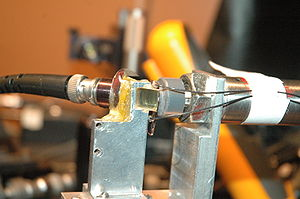# Attenuated Total Reflectance

### Overview

Attenuated Total Reflection or ATR is a technique used together with Teng Mann to measure the R33 of electro-optic materials. A beam of IR light is directed through a prism at an angle exceeding the critical angle for internal reflection. This produces an evanescent wave at the prism surface. If a EO polymer is pressed in intimate contact with the prism they can be coupled so that he evanescent wave stimulates emission from the sample. This emission is measured with a photodiode. An electric field applied to an EO polymer changes its index of refraction which alters the critical angle.

Attenuated Total Reflection simulation

<swf width="720" height="480">http://depts.washington.edu/cmditr/media/atr.swf</swf>

The above simulation allows you visualize what is happening during ATR measurement.

The EO material sample is pressed against the prism by the pneumatic plunger. The angle of the prism with respect to the laser is controlled with the slide bar. 0 degrees means the edge of the prism is normal to the laser beam and the sample is 45 degrees from the beam.

The photodiode produces a current when there is reflection from the sample. At certain critical angles the sample traps light due to total internal reflection and its reflectance is attenuated.

The field strength to the sample is controlled with the other slide 0-10 volts. This controls the EO effect in the sample.

Click Auto graph to record the diode current and the sample lockin voltage across a range of angles from -12 to 0 ( -2800 to 0 microsteps). Repeat this measurement at various field strengths to see the effect on the diode current.

### Significance

The electro-optic coefficient for a poled polymer film can be calculated as follows

$r_{33} = \frac {2d\Delta R} {n^3_{TM} V_m} \frac {\delta n_{eff}} {\delta \theta} \left /( \frac {\delta R \delta n_{eff} } {\delta \theta \delta n_{TM} } \right ) \,\!$

where

$R\,\!$ is the DC reflected signal
$V_m\,\!$ is the AC modulation voltage
$n_{eff}\,\!$ is the ordinary index of refraction
$n_{TM}\,\!$ is the film refractive index

### TechniqueIn the prism coupling technique the sample is pressed between a prism and light pipe connected to a detector. The "wet spot" is the point where the light pipe is in perfect contact with the sample so they behave as a single unit.